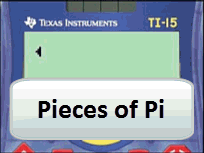# Activities

•• ##### Subject Area

• Standard: National Curriculum 7-10: Measurement and Geometry: Shape
• Standard: National Curriculum 7-10: Measurement and Geometry: Using units of measurement

• ##### AuthorPrimary
Middle

120 Minutes

• ##### Device
• TI-15 Explorer™

## Pieces of Pi#### Activity Overview

Students measure the circumference and diameter of circular objects. The measurements are used to estimate pi. A rule is established and entered into the calculator to predict the circumference of other circles.

#### Objectives

Investigate index notation and represent whole numbers as products of powers of prime numbers. ACMMG-197

#### Vocabulary

• Circumference
• Radius
• Diameter

#### About the Lesson

Students measure the circumference and diameter of circular objects, tabulate the data and determine the ratio between circumference and diameter of a circle. (pi) In the second part of this task students use this ratio in a formula to determine the circumference of a circle.
This activity includes teacher notes and curriculum references, two worksheets, an assessment task and suggested answer.